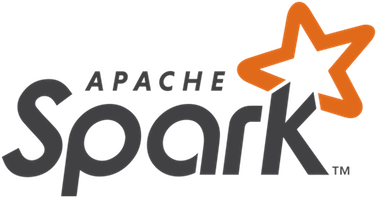Lightning-fast unified analytics engine

Archive

## Apache Spark Examples

These examples give a quick overview of the Spark API. Spark is built on the concept of distributed datasets, which contain arbitrary Java or Python objects. You create a dataset from external data, then apply parallel operations to it. The building block of the Spark API is its RDD API. In the RDD API, there are two types of operations: transformations, which define a new dataset based on previous ones, and actions, which kick off a job to execute on a cluster. On top of Spark’s RDD API, high level APIs are provided, e.g. DataFrame API and Machine Learning API. These high level APIs provide a concise way to conduct certain data operations. In this page, we will show examples using RDD API as well as examples using high level APIs.

## RDD API Examples

### Word Count

In this example, we use a few transformations to build a dataset of (String, Int) pairs called `counts` and then save it to a file.

### Pi Estimation

Spark can also be used for compute-intensive tasks. This code estimates π by "throwing darts" at a circle. We pick random points in the unit square ((0, 0) to (1,1)) and see how many fall in the unit circle. The fraction should be π / 4, so we use this to get our estimate.

## DataFrame API Examples

In Spark, a DataFrame is a distributed collection of data organized into named columns. Users can use DataFrame API to perform various relational operations on both external data sources and Spark’s built-in distributed collections without providing specific procedures for processing data. Also, programs based on DataFrame API will be automatically optimized by Spark’s built-in optimizer, Catalyst.

### Text Search

In this example, we search through the error messages in a log file.

### Simple Data Operations

In this example, we read a table stored in a database and calculate the number of people for every age. Finally, we save the calculated result to S3 in the format of JSON. A simple MySQL table "people" is used in the example and this table has two columns, "name" and "age".

## Machine Learning Example

MLlib, Spark’s Machine Learning (ML) library, provides many distributed ML algorithms. These algorithms cover tasks such as feature extraction, classification, regression, clustering, recommendation, and more. MLlib also provides tools such as ML Pipelines for building workflows, CrossValidator for tuning parameters, and model persistence for saving and loading models.

### Prediction with Logistic Regression

In this example, we take a dataset of labels and feature vectors. We learn to predict the labels from feature vectors using the Logistic Regression algorithm.